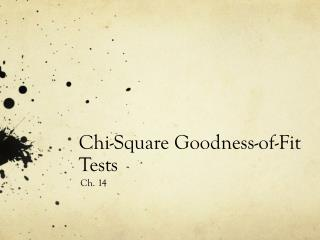DownloadDownload PresentationChi-Square Goodness-of-Fit Tests

# Chi-Square Goodness-of-Fit Tests

Télécharger la présentation## Chi-Square Goodness-of-Fit Tests

- - - - - - - - - - - - - - - - - - - - - - - - - - - E N D - - - - - - - - - - - - - - - - - - - - - - - - - - -
##### Presentation Transcript

1. One-way Table One way tables display the distribution of a categorical variable for the individuals in a sample.

2. Hypotheses Ho: The company’s stated color distribution for M&M’S Milk Chocolate Candies is correct Ha: The company’s stated color distribution for M&M’s Milk Chocolate Candies is not correct Ho: pblue = .24, porange = .20, pgreen = .16, pyellow = .14, pred = .13, pbrown = .13 Ha: At least two of the pi’s is incorrect

3. Observed Counts vs. Expected Counts Observed counts – the counts you get from your sample Expected counts – the counts you would expect to get from your sample if Ho is true.

4. Expected Counts for M&M’S

5. Chi-square Statistic The chi-square statistic is a measure of how far the observed counts are from the expected counts. The formula for the statistic is where the sum is over all possible values of the categorical variable

6. Calculate the Chi-Square Statistic

7. Check for Understanding Mars, Inc., reports that their M&M’S Peanut Chocolate Candies are produced according to the following color distribution: 23% each of blue and orange, 15% each of green and yellow and 12% each of red and brown. You bought a bag of Peanut M&M’S and counted the colors of the candies in your sample: 12 blue, 7 orange, 13 green, 4 yellow, 8 red, and 2 brown. • State the appropriate hypotheses for testing the company’s claim about the color distribution. • Calculate the expected count for each color. • Calculate the chi-square statistic for your sample.

8. The Chi-Square Distributions If we simulated taking 500 random samples of 60 M&M’s Milk Chocolate Candies and calculated the χ2 statistic for each and then created a dot plot of the 500 χ2. This would be a sampling distribution. This sampling distribution would be close to a chi-square distribution with degrees of freedom n – 1.

9. The Chi-Square Distributions The chi-square distributions are a family of distributions that take only positive values and are skewed to the right. A particular chi-square distribution is specified by giving its degrees of freedom. The chi-square goodness-of-fit test uses the chi-square distribution with degrees of freedom = the number of categories – 1

10. Finding the P-value In our M&M’s example χ2 = 10.18. Our degrees of freedom (df) = 6-1, df = 5. Using the chi-square distribution critical values table (table D) find the P-value interval.

11. Finding the P-value(on your calculator) On your calculator χ2cdf(χ2 statistic, large number, df) You find χ2cdf under distributions. Now find the exact P-value for our M&M’s example. Should we reject Ho?

12. Large Sample Size Condition The chi-square goodness-fit-test uses some approximations that become more accurate as we take more observations. One rule of thumb is that all expected counts must be at least 5. This Large Sample Size condition takes the place of the Normal condition for z and t procedures. You still need to check that the Random and Independent conditions are met.

13. The Chi-Square Goodness-of-Fit Test Suppose the Random, Large Sample Size, and Independent conditions are met. To determine whether a categorical variable has a specified distribution, expressed as the proportion of individuals falling into each possible category, perform a test of Ho: The specified distribution of the categorical variable is correct Ha: The specified distribution of the categorical variable is not correct. We can also write these hypotheses symbolically. Ho: p1 = ___, p2 = ____,… pi = _____ Ha: At least one of the pi’s is incorrect

14. The Chi-Square Goodness-of-Fit Test Start by finding the expected count for each category assuming that Ho is true. Then calculate the chi-square statistic. where the sum is over all the categories. The P-value is the area to the right of χ2 under the density curve of the chi-square distribution with n – 1 degrees of freedom.

15. The Conditions Random – the data come from a random sample or a randomized experiment Large Sample Size – All expected counts are at least 5 Independent – Individual observations are independent. When sampling without replacement, check that the population is at least 10 times as large as the sample.

16. Cautions • The chi-square test statistic compares observed and expected counts. Don’t try to perform calculations with the observed and expected proportions in each category. • When checking the Large Sample Size condition, be sure to examine the expected counts, not the observed counts.

17. When were you born? Are births evenly distributed across the days of the week? The one-way table below shows the distribution of births across the days of the week in a random sample of 140 births from local records in a large city: Do these data give significant evidence that local births are not equally likely on all days of the week? Use α = .05.

18. Chi-Square Goodness-of-Fit Test on Calculator • Enter the observed counts and expected counts into two lists. • Find χ2GOF – Test … under Stat tests • You will be given χ2, P-value, df and CNTRB or CompList • CNTRB or CompList show which terms contribute most to the chi-square statistic.